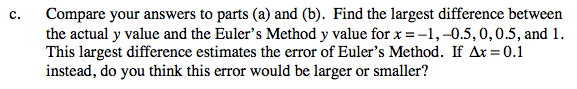### Home > CALC > Chapter 7 > Lesson 7.4.3 > Problem7-196

7-196.
1. y = f(x) satisfies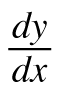= 2x and its graph contains the point (−1, 5). Homework Help ✎

1. Use Euler' s Method to sketch y for −1 ≤ x ≤ 1. Use Δx = 0.5.

2. Use implicit integration to find y.

3. Compare your answers to parts (a) and (b). Find the largest difference between the actual y value and the Euler's Method y value for x = − 1, −0.5, 0, 0.5, and 1. This largest difference estimates the error of Euler's Method. If Δx = 0.1 instead, do you think this error would be larger or smaller?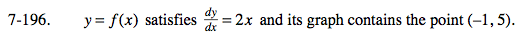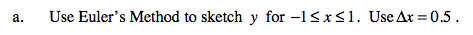Show all steps and carefully record the coordinate points at x = −0.5, x = 0, x = 0.5 and x = 1.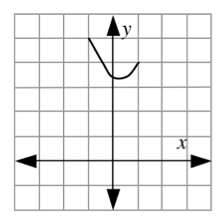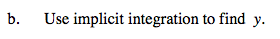Use (−1, 5) to help you find the value of C after you integrate.